# Resistor Color Code Calculator

Created by Hanna Pamuła, PhD candidate
Reviewed by Dominik Czernia, PhD candidate
Last updated: Aug 06, 2021

With this resistor color code calculator you'll quickly and easily find out the resistance of your element. Just choose how many bands does your resistor have - 4, 5 or 6, select the colors, and in the blink of an eye you'll get the resistance with tolerance, range and temperature coefficient value (if you've chosen 6 band resistor color code). If you want to understand how to read resistor color code, scroll down and you'll find there formulas and explanation. Also, we show 10k resistor color code as well as many other informative examples.

## How to read resistor color code?

Color bands are an easy and cheap way of indicating the value of an electronic components. On the tiniest resistors the printed alphanumeric codes would be too small to read, so the color code was developed in the early 1920s.

The first question which usually arises is: how do I know from which end should I start reading my resistor color code?
Fortunately, couple visual hints exist!

• In a usual case, the bands are not spaced regularly - there's a gap, and bands are somehow grouped. The larger gap occurs before the tolerance band. Put the bigger group on the left side and read resistors from left to right.
• Very often the tolerance of the resistor is equal to 5% or 10%. These values are marked with metallic colors - gold and silver, respectively. However, the resistor color code never starts from such color - so if you find the metallic color on your resistor, it's definitely the tolerance value, so it must be placed on the right side. Again, read the resistor from left to right.
• Usually, the first band will be closest to the end (but not always, so then use other clues).

If none of the above appear to help your problem, you can always use a multimeter to tell between two possible resistances - and reading directions.

Ok, let's get down to brass tacks: How to read resistor color code?

The value of the resistance is marked with the colors. Every color is a different number:

color name digit color color name digit color
black 0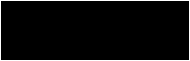green 5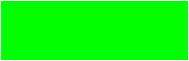brown 1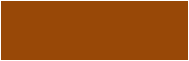blue 6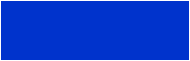red 2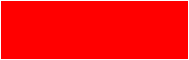violet 7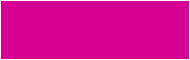orange 3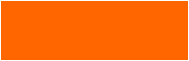gray 8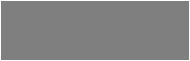yellow 4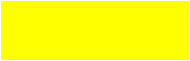white 9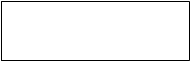It's the color code working for the first 2 or 3 bands from the left side.

Then, we have the band called multiplier, and the colors meaning is different:

color name multiply color color name multiply color
black x1Ωblue x1MΩbrown x10Ωviolet x10MΩred x100Ωgray x100MΩorange x1kΩwhite x1GΩyellow x10kΩgold x0.1Ω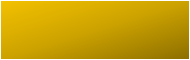green x100kΩsilver x0.01Ω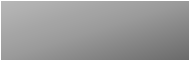Here, color represents the power of 10, by which the number created from previous bands must be multiplied. You can express the multipliers with prefixes like kilo, mega or giga (kΩ, MΩ, GΩ), but also the scientific notation is used - e.g. 10⁹Ω (gigaohm).

And finally, the last band which occurs in all types of resistors - 4, 5 and 6 band - is a tolerance band. It's expressed in percentages, and the variation in components resistance is mostly of a statistical nature (normal distribution):

color name tolerance color color name tolerance color
brown ±1%violet ±0.1%red ±2%gray ±0.05%green ±0.5%gold ±5%blue ±0.25%silver ±10%So that's all you need to know about the colors meaning for 4 and 5 band resistor color codes. For 6 band there's additional ring indicating the temperature coefficient - read more about it in a paragraph dedicated to 6 band resistors. Scroll down and find out the formulas, depending on the type of your resistor!

## Example on how to use this resistor color code calculator

We've tried really hard to make the resistor color code calculator as simple and intuitive as possible, but if you have any problems, just have a look at the example below!

1. Choose the number of bands on your resistor. There are three options: 4, 5 or 6 bands. Let's assume you have a resistor with five bands.
2. Pick the colors of the bands. If you don't know which is the first and which is the last band, have a look at the pictures built-in the calculator. Generally, there's a gap before the tolerance band, so that's how you can recognize start and end. In our example, let's say we have the colors: brown, red, violet, black and red.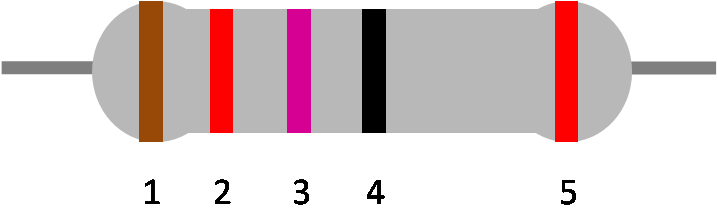1. The calculator draws the colored band. Compare them with your resistor, is it the same order?
2. When you finish entering all bands, the resistor color code calculator will show you the resistance, with tolerance and maximum and minimum value resulting from a tolerance. In our example, the resistance should be equal to 127Ω. Additionally, if you've entered 6 band resistor color code, the 6th band meaning will be displayed as well: the temperature coefficient, in ppm/°C.

Now, as you know how to read resistor color code, maybe you'd like to make circuit with resistors in parallel or in series? We have also other tools which are closely linked to the topic, like calculation of wire resistance or LED resistor calculator determining what resistance you should use when creating electronic circuit with LEDs. You can also check out our wheatstone bridge calculator.

## 4 band resistor color code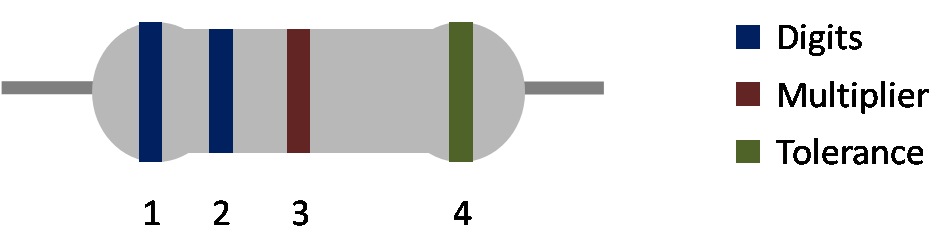The formula for 4 band resistor color code may be written as:

R = band3 * [(band1 * 10) + band2] ± band4

But what does it mean, how to read that? Let's have a look at the example and everything should be clear:

1. Assume we have a resistor with 4 color bands. The colors are: green, red, red and gold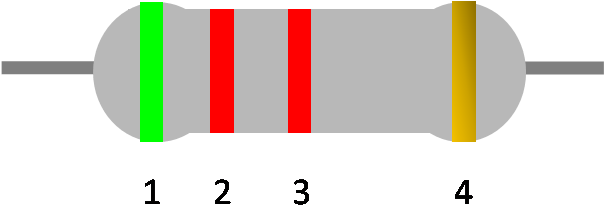1. Take the first two colors - green and red. The corresponding digits are 5 and 2. Put them together, and you'll get the number 52. You can write it formally as:

(band1 * 10) + band2 so in our case

(5 * 10) + 2 = 52

1. Take the third band - red. This time the meaning is different, because it's the multiplier band, and the corresponding factor is 100 Ω. Multiply the previous result by this value.

R = band3 * [(band1 * 10) + band2] so in our example:

R = 100Ω * [(5 * 10) + 2] = 5200Ω = 5.2kΩ

Here you go! That's your resistor value. But one band is left. And it's...

1. The tolerance band. In our case the band is gold, so the tolerance is equal to 5%. That means that our resistor value is not exactly the 5.2kΩ, but 5.2kΩ ± 5%. So the value may lay anywhere in the range <Rmin, Rmax>:
• minimum value: Rmin = R - band4 * R in our example:

Rmin = 5.2kΩ - 5.2kΩ * 5% = 5.2kΩ - 0.26kΩ = 4.94kΩ

• maximum value: Rmax = R + band4 * R so in our case:

Rmax = 5.2kΩ + 5.2kΩ * 5% = 5.2kΩ + 0.26kΩ = 5.46kΩ

And that's all! It wasn't so hard, was it? Check out the result with our resistor color code calculator.

## 5 band resistor color code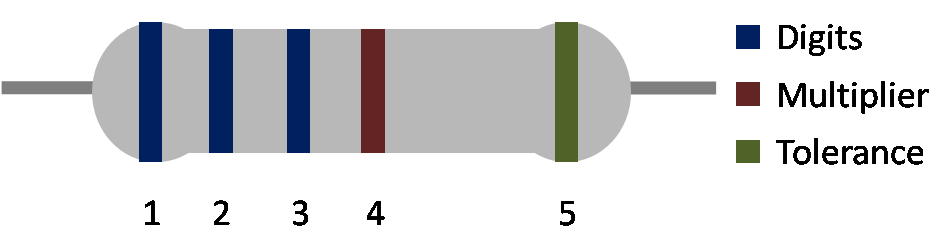The difference between 4 and 5 band resistors lays in significant digits - it's 2 or 3, respectively. So formula for 5 band resistor color code may be written as:

R = band4 * [(band1 * 100) + (band2 * 10) + band1] ± band5

Let's just expand our previous example - after two significant bands, green and red, let's put the blue one: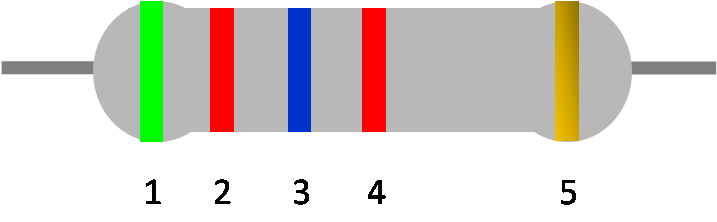1. For green, red and blue, the corresponding digits are 5, 2 and 6. It's our number - 526. Write it formally as:

(band1 * 100) + (band2 * 10) + band1 so in our case

(5 * 100) + (2 * 10) + 6 = 526

1. Fourth red band is our multiplier ring again, with the corresponding factor of 100 Ω. Multiply obtained result by this value:

R = band4 * [(band1 * 100) + (band2 * 10) + band1] so in our example:

R = 100Ω * [(5 * 100) + (2 * 10) + 6] = 52600Ω = 52.6kΩ

1. And finally, gold tolerance band means tolerance of 5%. Our resistor may lay anywhere in the range <Rmin, Rmax>:
• minimum value: Rmin = R - band5 * R so in our example:

Rmin = 52.6kΩ - 5.26kΩ * 5% = 52.6kΩ - 2.63kΩ = 49.97kΩ

• maximum value: Rmax = R + band5 * R in our case:

Rmax = 52.6kΩ + 52.6kΩ * 5% = 52.6kΩ + 2.63kΩ = 55.23kΩ

## 6 band resistor color code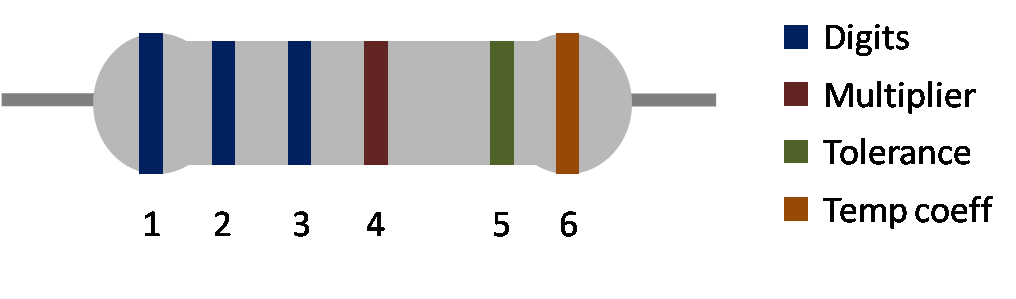6 band resistor color code is almost like 5 band resistor, but it additionally includes a temperature coefficient band at last position. This Thermal Coefficient (TCR) defines the change in resistance as a function of the ambient temperature and it's expressed in ppm/°C. For example, if we have a resistor with TCR equal to 50 ppm/°C, then it means that the resistance won't change more than 0.00005 ohms per ohm per degree Centigrade temperature change (but only in referenced temperature range, check out the element's manual). Given TCR and information that resistor's initial value in room temperature T0 = 25°C is equal to e.g.R0 = 50Ω, we can calculate the resistance R after heating or cooling element to other temperature, e.g. T = 50°C:

R = R0 * (1 + TCR * (T - T0))

R = 50Ω * (1 + 0.00005 1/°C * 25°C) = 50.0625Ω

For that calculations we can also use Kelvin instead of degree Centigrade temperature, as the difference between temperatures is the thing that matters, not the absolute temperature value. Similar concept to TCR is a Thermal Expansion Coefficient - here not the resistance, but the length or volume of the element changes with the temperature.

Watch out! Sometimes the sixth band doesn't mean the thermal coefficient but the reliability of the resistor, but those are sporadic cases.

The colors of the last band are coded as:

color name TCR [ppm/°C] color color name TCR [ppm/°C] color
brown 100yellow 25red 50blue 10orange 15violet 5## What's the 10k resistor color code?

There's a lot of options, depending on tolerance and number of bands.

• 4 band resistor color code for 10k resistor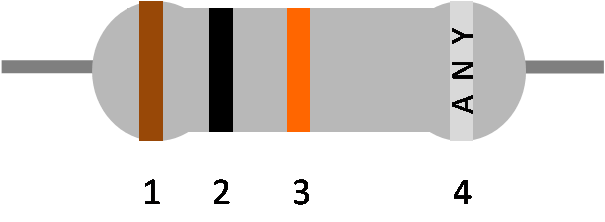Always first three bands are the same:

1. The first band is brown as it stands for 1
2. The second band is black which means 0
3. The third band - multiplier x 1 kΩ - is orange
4. The fourth band depends on the tolerance - so any color is possible for tolerance band

Just to quickly check the calculations:

R = [(band1 * 10) + band2)] * band3

R = [(1 * 10) + 0)] * 1kΩ = 10 * 1kΩ = 10kΩ

Yes! Looks good.

• 5 and 6 band resistor color code for 10k resistor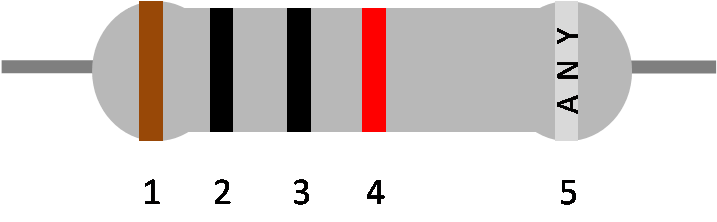Always first four bands are fixed:

1. The first band is brown as it stands for 1
2. The second band is black which means 0
3. The third band is black which means 0
4. The fourth band is a multiplier x 100Ω which is red
5. The fifth (and sixth) band may be different, as they're the tolerance and thermal coefficients values

Check out again:

R = [(band1 * 100) + (band2 * 10) + band3)] * band4

R = [(1 * 100) + (0 * 10) + 0)] * 100Ω = 100 * 100Ω = 10kΩ

It's working. Great!

Hanna Pamuła, PhD candidate
Number of bands
four
Bands colors
Band 1
Select...
Band 2
Select...
Multiplier
Select...
Tolerance
Select...People also viewed…

### Car center of mass

Use this car center of mass calculator and find the exact position of the center of gravity of your car.

### Car heat

The hot car calculator shows you how fast the interior of a car heats up during a summers day.

### Ideal gas pressure

Skip manual calculations for the pressure of an ideal gas using this ideal gas pressure calculator.

### Steps to calories

Steps to calories calculator helps you to estimate the total amount to calories burned while walking.